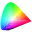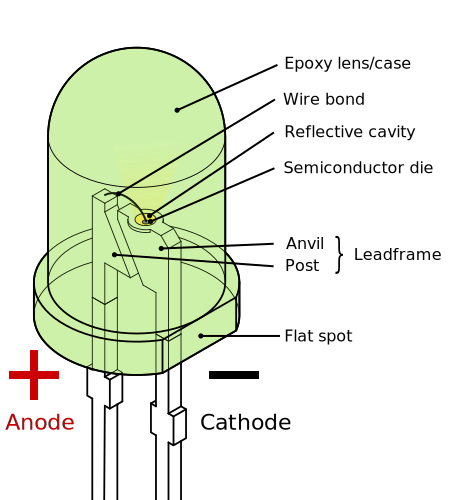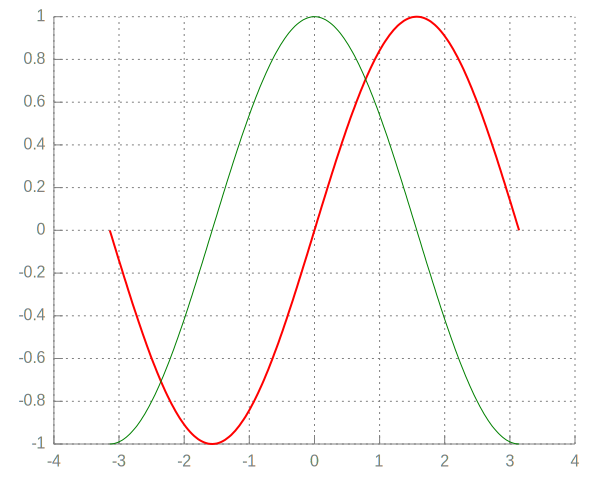# IPerl display demo

author: Zaki Mughal

date: 2015-02-09

View this notebook on nbviewer.

This notebook demonstrates how to use the rich display system in IPerl and how it can be extended on the fly.

All data that is displayed implements a Displayable role. This role requires a method called iperl_data_representations that returns a HashRef of different representations of the data where the keys are the MIME type of the data (e.g., text/html) and values are the strings that contain the bytestream for that MIME type. For example, with PNG, we have

  use v5.16;
use DDP; # Data::Printer

my $png_display = Devel::IPerl::Display::PNG->new( "http://www.libpng.org/pub/png/PngSuite/ccwn3p08.png" ); say &p( [ keys$png_display->iperl_data_representations ] );

$png_display; [  "image/png",  "text/html",  "text/plain" ]Notice that I just displayed that PNG by just putting the $png_display variable at the end? That's because any displayable is automatically displayed if it is at the end of a cell.

But there's a problem: I don't want to type or remember Devel::IPerl::Display::PNG every time I want to load up a PNG.

Instead, you can just call the helper method IPerl->png() and you'll get the same result.

  IPerl->png( "http://www.libpng.org/pub/png/PngSuite/ccwn3p08.png" );There are other Displayables too. For example, let's load up an <iframe>.

  my $iframe_display = IPerl->iframe( "http://metacpan.org/recent", width => "75%" ); What if we want to display multiple things in one cell? For example, we want to loop over a number of images and display each of them? You can do that by calling the IPerl->display() on the Displayable object.  my @svg = split ' ', q[ https://upload.wikimedia.org/wikipedia/commons/f/fd/Ghostscript_Tiger.svg https://upload.wikimedia.org/wikipedia/commons/f/f9/LED,_5mm,_green_(en).svg ]; IPerl->display( IPerl->svg($_ , width => "200" ) ) for @svg;

$png_display;There is also a way to display arbitrary HTML. Here, we create a simple HTML table that loops over a 2D nested ArrayRef. We could send the string directly to the IPerl->html() method, but that isn't very DRY. Instead, we'll create our own helper!  IPerl->helper( my_table => sub { my ($self, $data) = @_; return unless ref$data eq 'ARRAY';

my $html = "<table>"; for my$row (@$data) {$html .= "<tr>";
for my $cell (@$row) {
my $cell_html =$cell->iperl_data_representations->{"text/html"};
$html .= "<td>$cell_html</td>\n";
}
$html .= "</tr>"; }$html .= "</table>";

IPerl->html( $html ); }); my$N = 4; my $M = 10; my$d = [ ([ ( $png_display ) x$M ]) x $N ]; IPerl->my_table($d  );There are other plugins besides the Displayables that work directly on file types (PNG, SVG, HTML, etc.).

For example, you can load a plugin that adds a role to PDL::Graphics::Gnuplot and makes it displayable as an SVG.

  IPerl->load_plugin( "PDLGraphicsGnuplot" );
use PDL;
use PDL::Graphics::Gnuplot;
use PDL::Constants qw(PI);

my $gp = gpwin(); # do some styling$gp->option( topcmd => <<'GP');
# define axis
# remove border on top and right and set color to gray
set style line 11 lc rgb '#808080' lt 1
set border 3 back ls 11
set tics nomirror

# define grid
set style line 12 lc rgb '#808080' lt 0 lw 1
set grid back ls 12
GP

my $theta = zeros(200)->xlinvals(-1*PI, 1*PI);$gp->plot( { lw => 2 }, $theta, sin($theta), {}, $theta, cos($theta)  );

IPerl->display( IPerl->tex( q| $\sin\theta$ and $\cos\theta$ | ) );

\$gp;Have fun and let me know what you make with your IPerl notebooks!